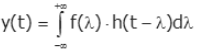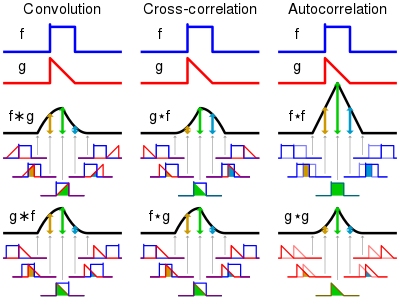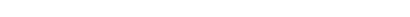## How do I implement the convolution integral of 2 c...

Hi there!

I'm attempting to develop a custom library in MapleSim (a Modelica solver engine) that can use convolution integrals to model the hydrodynamic behaviour of floating bodies. The convolution integral is mathematically represented as follows:And the equation I'm particularly interested in solving is as follows:I'm trying to solve this convolution integral in Modelica using a model that can be imported to MapleSim. So far, I've had no luck in implementing this convolution for continuous functions symbolically. I have used a numerical approach on 2 arrays using the following approach in Modelica:

``````// Modelica function
function convIntegral
input Real simTime;  // Simulation time
input Real f[:];     // Kernel function array
input Real g[:];     // Second function array

output Real h[(2*simTime) - 1];    // Output the convolution integral in the form of an array

// Define the algorithm to numerically compute the convolution integral of 2 arrays
algorithm
// Initialize the output array with zeroes
for i in 1:((2*simTime) - 1) loop
h[i] := 0;
end for;

// Iterate over the simulation time
// Recursively increment the convolution array with the pointwise product of the 2 functions
for i in 1:simTime loop
for j in 1:simTime loop
h[i+j-1] := (f[i] * g[j]) + h[i+j-1];
end for;
end for;
end convIntegral;
// End of function to compute the convolution integral``````

This works perfectly for discrete samples and I have verified it with output from Matlab's inbuilt function

``conv(A,B)  % For 2 arrays A and B``

However, I would like to implement this on 2 continuous functions and this numerical approach does not work since MapleSim does not support conversion between discrete and continuous signals.

I understand that convolution is essentially an operation between two functions, where we time-flip one of the functions (kernel) and then slide it across the other function while measuring the bounded area and outputting that as the result of the convolution. I include this image from Wikipedia that sums up convolution: (Not including links as they mark questions as spam)I've tried implementing this in Modelica using the following code:

``````// Model to perform convolution on 2 continuous functions
model ConvolutionalIntegral

extends Modelica.Blocks.Icons.Block;

// Define model variables
Real kernelFunction = (e ^ (-0.5 * time)) * cos(0.5 * time);  // I've taken an example of a function I might use
Real kernelFunctionFlipped = (e ^ (-0.5 * (T_sim - time))) * cos(0.5 * (T_sim - time));  // I've flipped the kernel function about the vertical axis by converting the (time) variable to (T_sim - time) where (T_sim) is a variable storing the simulation duration
Real secondFunction;  // The other function for the convolution
Real convolutionIntegralOutput;  // Function to store the output

equation
// Convolution implementation
der(convolutionIntegralOutput) = kernelFunctionFlipped * secondFunction;

// Final equation to solve
der(secondFunction) + convolutionIntegralOutput = 0;
// An example of a differential equation I'd like to solve involving the convolution integral

end ConvolutionIntegral;``````

I had hoped that this would yield the output of the convolution since I'm essentially multiplying the time-flipped kernel and the other function and then integrating them over time. However, the output does not provide the expected result and it appears that Modelica interprets my code to mean that I'm integrating the pointwise product of these 2 functions over time instead of sliding the kernel over the other function.

I'd appreciate it if you could take a look at my code and my approach to solving the convolution integral symbolically, and point out where I'm making a mistake and what a possible fix might be.

Thank you!

## How do I implement expandable connectors from Mode...

Hi there!

I'm working on implementing a custom Modelica Library in MapleSim 2021. I have Maple 2021 installed and my software is up to date. The library I have developed is in a single file (extension ".mo") which I developed on an IDE for Modelica i.e., I did not create the library using MapleSim. During the import into MapleSim, no errors appear in the system logs. All my components and models have been imported except for an "expandable connector". It appears that the problem is with the term "expandable".

Since this expandable connector does not appear among my library components, I attempted to create a custom component using the Modelica code editor in MapleSim. However, the file cannot be saved while I prefix the term "expandable" to "connector". The software allows me to save the file with the new code after dropping the "expandable" term.

I know that expandable connectors are used by Modelica. Here are the references I used during development:

https://mbe.modelica.university/components/architectures/expandable/

Working with Expandable Connectors - Claytex

However, there does not seem to be any information on expandable connectors in MapleSim. I'd appreciate it if any of you could throw some light on why I'm not able to import this component into MapleSim and fixes/suggestions on what I might be doing wrong. If any further information on my question is required, please do let me know.

## Critical fix for Maplesoft products on macOS

Maple 2021 MapleSim 2021 Maple Flow 2021

We have just issued a critical fix to Maple, MapleSim, and Maple Flow running on macOS.

We have heard from some users who were experiencing serious problems with doubled characters while using Maplesoft products on macOS, including these reports on MaplePrimes. Further investigation determined that these problems appear specifically on macOS 11 and macOS 12.  I am happy to report that we have now corrected the problem, and a patch is available.

Anyone who uses macOS 11 or macOS 12 should install this update immediately. We also strongly recommend that all macOS users install this update, to avoid problems that may be triggered by future updates to your operating system.

To obtain this update:

For those who have experienced problems, we apologize for the inconvenience and thank you for your patience while we worked to find a solution.

## flange and frame definitions ...

Hi All!  New to MapleSIM and working on a program help my FIRST Robotics team start using MapleSIM.  I am looking for any presentation material already created, directed at high school kids.

Appreciate any links to presentation material I can repurpose for them.

Cheers,

Andy

## Copy & Paste in MapleSim not working...

Copying and Pasting of parameters (entered data) or text in text boxes does not work on my MapleSim 2021.1 Windows10 installation.

I tired the usual keys and context menus. Cutting works.

Thats what I get back when I paste text here after cutting

<math xmlns='http://www.w3.org/1998/Math/MathML'>[/itex]

Copy&Paste of components works.

Is this a known issue?